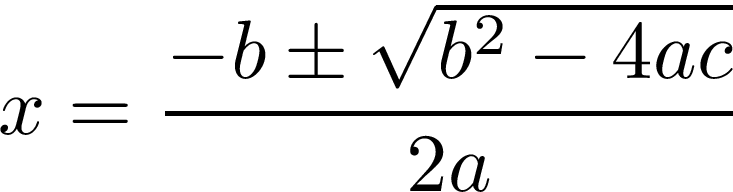The quadratic formula is used for solving quadratic equations. The actual creation of this formula is somewhat complex. Creating it requires the use of completing the square as well as square root property. Below is what the equation looks like.For our purposes, we will go through an example that solves a quadratic equation using the quadratic formula. In addition, we will also explore the idea of the discriminant as it relates to quadratic formulas.

Example

The mechanics of solving a quadratic formula using this approach is similar to most other methods. You simply plug in the substitutes in the equation to get your actual answer. Below is an example,We will now plug in the values and determine x.Discriminant

The discriminant of a quadratic equation is used to determine the type if the answer you would get if you solve the equation. THere are three types of answers that you can get when solving a quadratic equation.

• Two real solutions-This happens when the discriminant results are positive.
• One real solution-Happens when the discriminant results are zero
• Two complex-Happens when the discriminant is negative

A complex solution involves the use of an imaginary number. This happens when the square root number is negative, which is technically impossible. To deal with this in math the letter i is used instead of the negative sign below is an example.The actual formula for calculating the discriminant is already in the quadratic formula. You simply calculate only the information under the square root. This is shown below.IN our first example, we got two real solutions. We will now confirm this by calculating the discriminant.Are answer is positive, which means that we can expect to calculate to real solutions for this particular problem.

Conclusion

The quadratic formula provides another way to solve a quadratic equation. This is probably the easiest method to learn as it is simply a matter of plugging numbers into the formula. This may explain why the quadratic formula is frequently the first method algebra students learn for solving quadratic equations.

The discriminant is a shortcut calculation that allows you to determine the quality of the solutions you would get if you solve the equation.